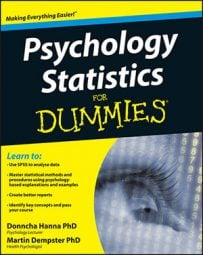##### Psychology Statistics For DummiesWhen working with psychology statistics you can classify variables according to their measurement properties. When you record variables on a data sheet, you usually record the values on the variables as numbers, because this makes statistical analysis easier. However, the numbers can have different measurement properties and these determine what types of analyses you can do with these numbers. The variable’s level of measurement is a classification system that tells you what measurement properties the values of a variable have.

The measurement properties that the values in a variable can possess are:

• Magnitude: This means that you can order the values in a variable from highest to lowest.

• Equal intervals: This means that a unit difference on the measurement scale is the same regardless of where that unit difference occurs on the scale.

• True absolute zero: The true absolute zero point means that the zero point on the measurement scale is the point where nothing of the variable exists and, therefore, no scores less than zero exist.

These three measurement properties enable you to classify the level of measurement of a variable into one of four types

• Nominal: This means that a variable has none of the three measurement properties. You measure a variable at the nominal level when you’re using the numbers in the variable only as labels.

• Ordinal: If you measure a variable at the ordinal level then the values on the variable have the measurement property of magnitude only. You measure a variable at the ordinal level when the scores in the variable are ordered ranks.

• Interval: If you measure a variable at the interval level of measurement, it has the measurement properties of magnitude and equal intervals

• Ratio: if you measure a variable at the ratio level of measurement, it has the measurement properties of magnitude, equal intervals and a true absolute zero.